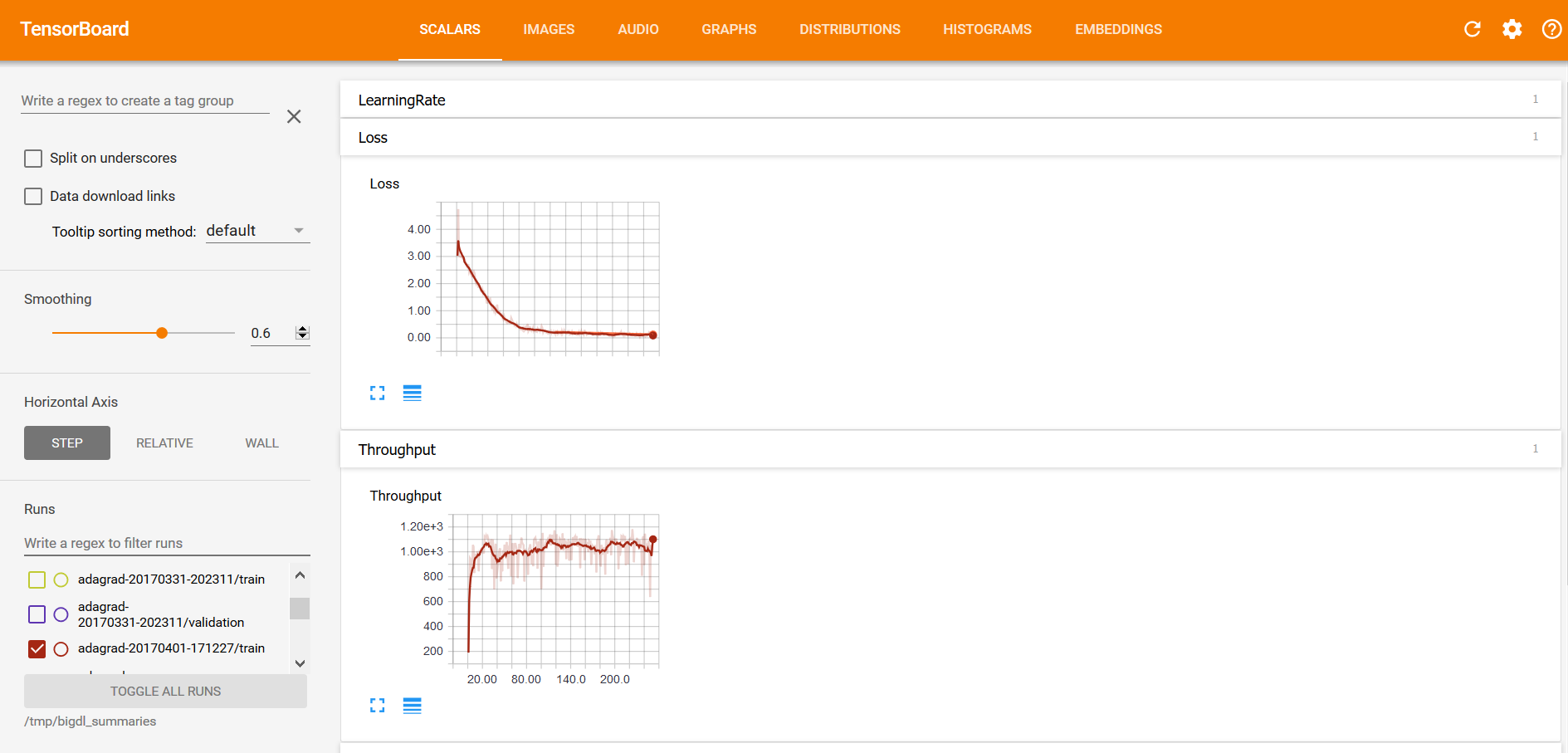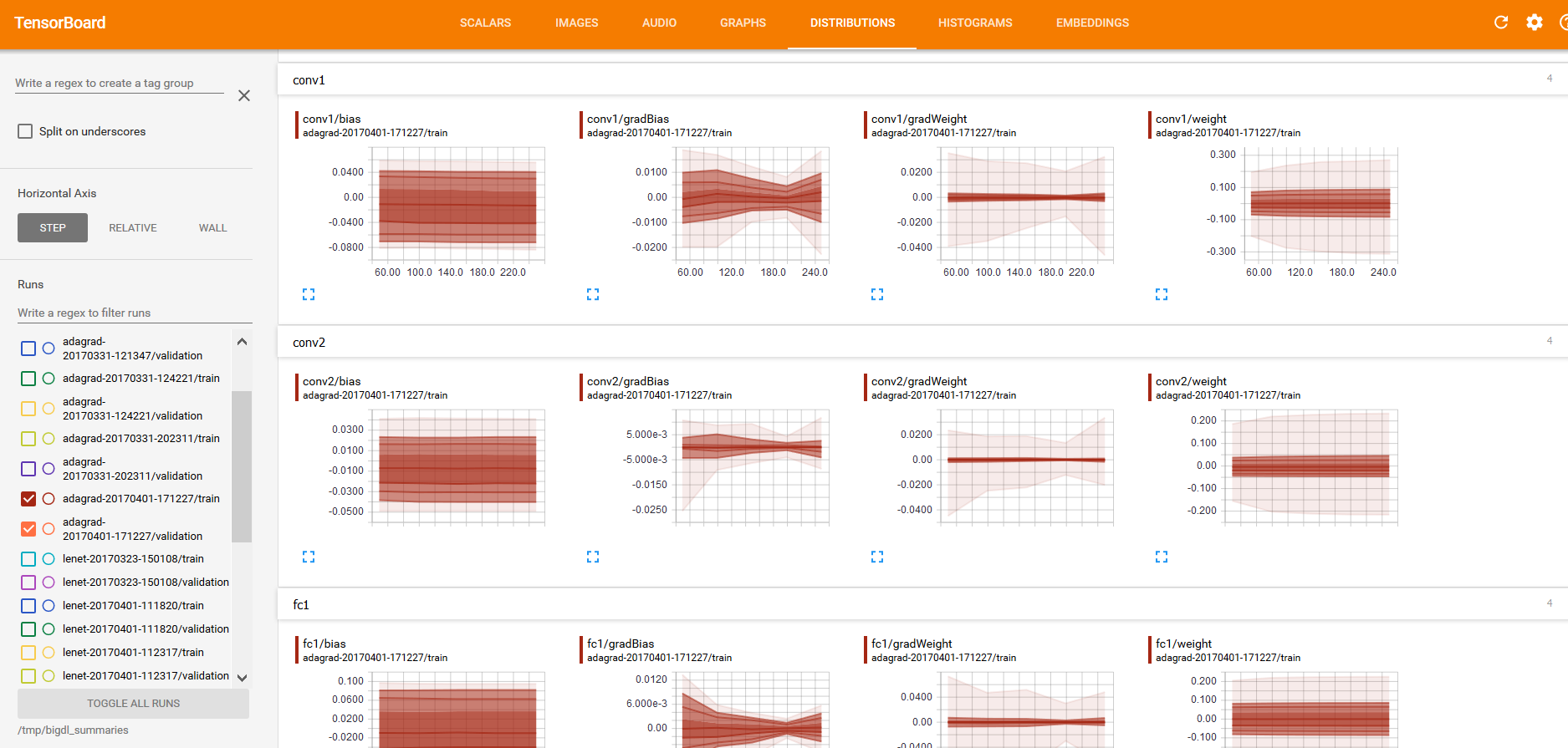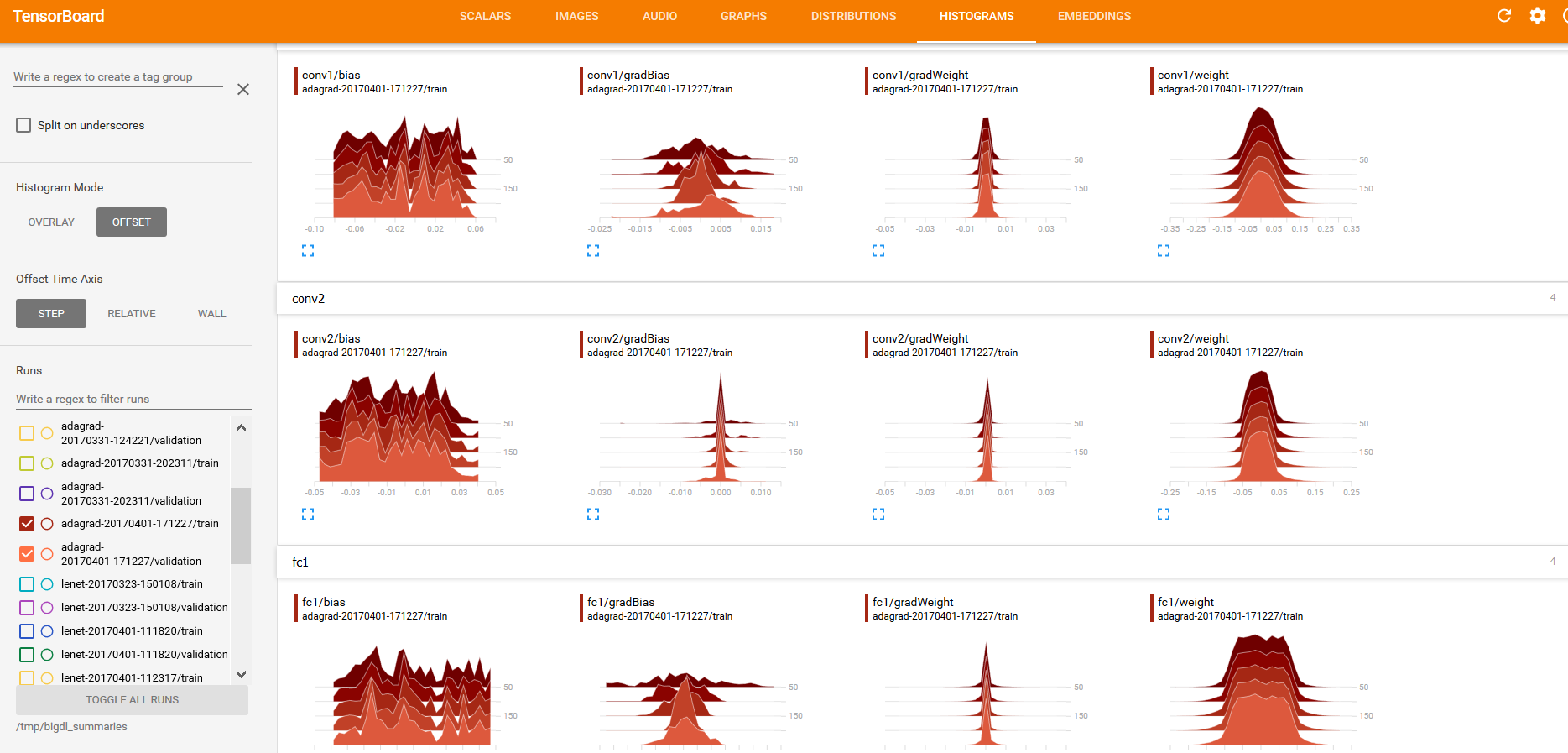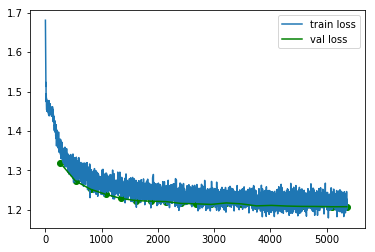# Visualization

## Generating summary info in BigDL

To enable visualization support, you need first properly configure the `Optimizer` to collect statistics summary in different stages of training (i.e. training (`TrainSummary`) and validation (`ValidationSummary`),respectively). It should be done before the training starts (calling `Optimizer.optimize()`). See examples below:

Example: Generating summary info in Scala

``````val optimizer = Optimizer(...)
...
val logdir = "mylogdir"
val appName = "myapp"
val trainSummary = TrainSummary(logdir, appName)
val validationSummary = ValidationSummary(logdir, appName)
optimizer.setTrainSummary(trainSummary)
optimizer.setValidationSummary(validationSummary)
...
val trained_model = optimizer.optimize()
``````

Example: Configure summary generation in Python

``````optimizer = Optimizer(...)
...
log_dir = 'mylogdir'
app_name = 'myapp'
train_summary = TrainSummary(log_dir=log_dir, app_name=app_name)
val_summary = ValidationSummary(log_dir=log_dir, app_name=app_name)
optimizer.set_train_summary(train_summary)
optimizer.set_val_summary(val_summary)
...
trainedModel = optimizer.optimize()
``````

After you start to run your spark job, the train and validation summary will be saved to `mylogdir/myapp/train` and `mylogdir/myapp/validation` respectively (Note: you may want to use different `appName` for different job runs to avoid possible conflicts.)

### Save graph model to summary so visualize model in tensorboard

Model structure is very important for people to create/understand model. For sequential models, you can just print them out by using the `toString` method. For complex graph model, you can use tensorboard to visualize it.

Here's how to save your graph model to summary log path to display it in the tensorboard.

Example: Save graph model to summary in Scala

``````val model = Graph(...)
model.saveGraphTopology("logpath")
``````

Example: Save graph model to summary in Python

``````model=Model(...)
model.save_graph_topology("logpath")

``````

## Retrieving summary info as readable format

You can use provided API `readScalar`(Scala) and `read_scalar`(Python) to retrieve the summaries into readable format, and export them to other tools for further analysis or visualization.

Example: Reading summary info in Scala

``````val trainLoss = trainSummary.readScalar("Loss")
val validationLoss = validationSummary.readScalar("Loss")
...
``````

Example: Reading summary info in Python

``````loss = np.array(train_summary.read_scalar('Loss'))
valloss = np.array(val_summary.read_scalar('Loss'))
...
``````

## Visualizing training with TensorBoard

With the summary info generated, we can then use TensorBoard to visualize the behaviors of the BigDL program.

• Installing TensorBoard

Prerequisites:

1. Python verison: 2.7, 3.4, 3.5, or 3.6
2. Pip version >= 9.0.1

To install TensorBoard using Python 2, you may run the command:

``````pip install tensorboard==1.0.0a4
``````

To install TensorBoard using Python 3, you may run the command:

``````pip3 install tensorboard==1.0.0a4
``````

Please refer to this page for possible issues when installing TensorBoard.

• Launching TensorBoard

You can launch TensorBoard using the command below:

``````tensorboard --logdir=/tmp/bigdl_summaries
``````

After that, navigate to the TensorBoard dashboard using a browser. You can find the URL in the console output after TensorBoard is successfully launched; by default the URL is http://your_node:6006

• Visualizations in TensorBoard

Within the TensorBoard dashboard, you will be able to read the visualizations of each run, including the “Loss” and “Throughput” curves under the SCALARS tab (as illustrated below):And “weights”, “bias”, “gradientWeights” and “gradientBias” under the DISTRIBUTIONS and HISTOGRAMS tabs (as illustrated below):## Visualizing training with Jupyter notebook

If you're using Jupyter notebook, you can also draw the training curves using popular plotting tools (e.g. matplotlib) and show the plots inline.

First, retrieve the summaries as instructed in Retrieve Summary. The retrieved summary is a list of tuples. Each tuple is a recorded event in format (iteration count, recorded value, timestamp). You can convert it to numpy array or dataframe to plot it. See example below:

Example: Plot the train/validation loss in Jupyter

``````#retrieve train and validation summary object and read the loss data into ndarray's.
loss = np.array(train_summary.read_scalar("Loss"))
val_loss  = np.array(val_summary.read_scalar("Loss"))

#plot the train and validation curves
# each event data is a tuple in form of (iteration_count, value, timestamp)
plt.plot(loss[:,0],loss[:,1],label='train loss')
plt.plot(val_loss[:,0],val_loss[:,1],label='val loss',color='green')
plt.scatter(val_loss[:,0],val_loss[:,1],color='green')
plt.legend();
``````## Logging

BigDL also has a straight-forward logging output on the console along the training, as shown below. You can see real-time epoch/iteration/loss/ throughput in the log.

``````  2017-01-10 10:03:55 INFO  DistriOptimizer\$:241 - [Epoch 1 0/               5000][Iteration 1][Wall Clock XXX] Train 512 in   XXXseconds. Throughput    is XXX records/second. Loss is XXX.
2017-01-10 10:03:58 INFO  DistriOptimizer\$:241 - [Epoch 1 512/             5000][Iteration 2][Wall Clock XXX] Train 512    in XXXseconds. Throughput   is XXX records/second. Loss is XXX.
2017-01-10 10:04:00 INFO  DistriOptimizer\$:241 - [Epoch 1 1024/            5000][Iteration 3][Wall Clock XXX] Train 512   in XXXseconds. Throughput    is XXX records/second. Loss is XXX.
``````

The DistriOptimizer log level is INFO by default. We implement a method named with `redirectSparkInfoLogs` in `spark/utils/LoggerFilter.scala`. You can import and redirect at first.

``````  import com.intel.analytics.bigdl.utils.LoggerFilter
LoggerFilter.redirectSparkInfoLogs()
``````

This method will redirect all logs of `org`, `akka`, `breeze` to `bigdl. log` with `INFO` level, except `org. apache.spark.SparkContext`. And it will output all `ERROR` message in console too.

You can disable the redirection with java property `-Dbigdl.utils. LoggerFilter.disable=true`. By default, it will do redirect of all examples and models in our code.

You can set where the `bigdl.log` will be generated with `-Dbigdl.utils. LoggerFilter.logFile=<path>`. By default, it will be generated under current workspace.